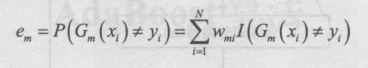python## 三、算法步骤1.初始化训练数据的权值分布，每一个训练样本最开始时都被赋予相同的权值 1/n2.进行多轮迭代，用 m = 1,2,…,k 表示迭代到第几轮

3.使用具有权值分布 Gm 的训练数据集学习，得到基本分类器4.计算 Gm(x) 在训练数据集上的分类误差率5.计算 Gm(x) 的系数，am表示 Gm(x) 在最终分类器中的重要程度6.更新训练数据集的权值分布，得到样本的新的权值分布，用于下一轮迭代7.组合各个弱分类器## 四、算法实现

```from numpy import *
import matplotlib.pyplot as plt

# 加载数据集
dataMat = []
labelMat = []
fr = open(fileName)
lineArr = []
curLine = line.strip().split('\t')
for i in range(numFeat - 1):
lineArr.append(float(curLine[i]))
dataMat.append(lineArr)
labelMat.append(float(curLine[-1]))
return dataMat, labelMat

# 返回分类预测结果  根据阈值所以有两种返回情况
def stumpClassify(dataMatrix, dimen, threshVal, threshIneq):
retArray = ones((shape(dataMatrix), 1))
if threshIneq == 'lt':
retArray[dataMatrix[:, dimen] <= threshVal] = -1.0
else:
retArray[dataMatrix[:, dimen] > threshVal] = -1.0
return retArray

# 返回 该弱分类器单层决策树的信息  更新D向量的错误率 更新D向量的预测目标
def buildStump(dataArr, classLabels, D):
dataMatrix = mat(dataArr)
labelMat = mat(classLabels).T
m, n = shape(dataMatrix)
numSteps = 10.0
bestStump = {}  # 字典用于保存每个分类器信息
bestClasEst = mat(zeros((m, 1)))
minError = inf  # 初始化最小误差最大
for i in range(n):  # 特征循环  （三层循环，遍历所有的可能性）
rangeMin = dataMatrix[:, i].min()
rangeMax = dataMatrix[:, i].max()
stepSize = (rangeMax - rangeMin) / numSteps  # (大-小)/分割数  得到最小值到最大值需要的每一段距离
for j in range(-1, int(numSteps) + 1):  # 遍历步长 最小值到最大值的需要次数
for inequal in ['lt', 'gt']:  # 在大于和小于之间切换
threshVal = (rangeMin + float(j) * stepSize)  # 最小值+次数*步长  每一次从最小值走的长度
predictedVals = stumpClassify(dataMatrix, i, threshVal,
inequal)  # 最优预测目标值  用于与目标值比较得到误差
errArr = mat(ones((m, 1)))
errArr[predictedVals == labelMat] = 0
weightedError = D.T * errArr
if weightedError < minError:  # 选出最小错误的那个特征
minError = weightedError  # 最小误差 后面用来更新D权值的
bestClasEst = predictedVals.copy()  # 最优预测值

bestStump['dim'] = i  # 特征
bestStump['thresh'] = threshVal  # 到最小值的距离 （得到最优预测值的那个距离）
bestStump['ineq'] = inequal  # 大于还是小于 最优距离为-1
return bestStump, minError, bestClasEst

# 循环构建numIt个弱分类器
weakClassArr = []  # 保存弱分类器数组
m = shape(dataArr)
D = mat(ones((m, 1)) / m)  # D向量 每条样本所对应的一个权重
aggClassEst = mat(zeros((m, 1)))  # 统计类别估计累积值
for i in range(numIt):
bestStump, error, classEst = buildStump(dataArr, classLabels, D)
alpha = float(0.5 * log((1.0 - error) / max(error, 1e-16)))
bestStump['alpha'] = alpha
weakClassArr.append(bestStump)  # 加入单层决策树

# 得到运算公式中的向量+/-α，预测正确为-α，错误则+α。每条样本一个α
# multiply对应位置相乘  这里很聪明，用-1*真实目标值*预测值，实现了错误分类则-，正确则+
expon = multiply(-1 * alpha * mat(classLabels).T, classEst)
D = multiply(D, exp(expon))  # 这三步为更新概率分布D向量 拆分开来了，每一步与公式相同
D = D / D.sum()

# 计算停止条件错误率=0 以及计算每次的aggClassEst类别估计累计值
aggClassEst += alpha * classEst
# 很聪明的计算方法 计算得到错误的个数，向量中为1则错误值
aggErrors = multiply(sign(aggClassEst) != mat(classLabels).T, ones((m, 1)))  # sign返回数值的正负符号，以1、-1表示
errorRate = aggErrors.sum() / m  # 错误个数/总个数
# print("错误率：", errorRate)
if errorRate == 0.0:
break
return weakClassArr, aggClassEst

# 预测 累加 多个弱分类器获得预测值*该alpha 得到结果
dataMatrix = mat(datToClass)
m = shape(dataMatrix)
aggClassEst = mat(zeros((m, 1)))
# 循环所有弱分类器
for i in range(len(classifierArr)):
# 获得预测结果
classEst = stumpClassify(dataMatrix, classifierArr[i]['dim'], classifierArr[i]['thresh'],
classifierArr[i]['ineq'])
# 该分类器α*预测结果 用于累加得到最终的正负判断条件
aggClassEst += classifierArr[i]['alpha'] * classEst  # 这里就是集合所有弱分类器的意见，得到最终的意见
return sign(aggClassEst)  # 提取数据符号

# ROC曲线，类别累计值、目标标签
def plotROC(predStrengths, classLabels):
cur = (1.0, 1.0)  # 每次画线的起点游标点
ySum = 0.0  # 用于计算AUC的值 矩形面积的高度累计值
numPosClas = sum(array(classLabels) == 1.0)  # 所有真实正例    确定了在y坐标轴上的步进数目
yStep = 1 / float(numPosClas)  # 1/所有真实正例 y轴上的步长
xStep = 1 / float(len(classLabels) - numPosClas)  # 1/所有真实反例 x轴上的步长
sortedIndicies = predStrengths.argsort()  # 获得累计值向量从小到大排序的下表index [50,88,2,71...]
fig = plt.figure()
fig.clf()
ax = plt.subplot(111)
# 循环所有的累计值 从小到大
for index in sortedIndicies.tolist():
if classLabels[index] == 1.0:
delX = 0  # 若为一个真正例，则沿y降一个步长，即不断降低真阳率；
delY = yStep  # 若为一个非真正例，则沿x退一个步长，尖笑阳率
else:
delX = xStep
delY = 0
ySum += cur  # 向下移动一次，则累计一个高度。宽度不变，我们只计算高度
ax.plot([cur, cur - delX], [cur, cur - delY], c='b')  # 始终会有一个点是没有改变的
cur = (cur - delX, cur - delY)
ax.plot([0, 1], [0, 1], 'b--')
plt.xlabel('False positive rate')
plt.ylabel('True positive rate')
plt.title('ROC curve for AdaBoost horse colic detection system')
ax.axis([0, 1, 0, 1])
plt.show()
print("the Area Under the Curve is: ", ySum * xStep)  # AUC面积我们以 高*低 的矩形来计算

# 测试正确率

errArr = mat(ones((67, 1)))  # 一共有67个样本
cnt = errArr[prediction10 != mat(testLabelArr).T].sum()
print(cnt / 67)

# 画出ROC曲线
classifierArray, aggClassEst = adaBoostTrainDS(datArr, labelArr, 10)
plotROC(aggClassEst.T, labelArr)
```

## 五、算法优化

• `权值更新方法的改进`

• `训练方法的改进`

• `多算法结合的改进`